Processing ......FreeComputerBooks.com Free Computer, Mathematics, Technical Books and Lecture Notes, etc.

Discrete and Finite MathematicsRelated Book Categories:
•Discrete Mathematics: An Open Introduction (Oscar Levin)

This is a gentle introduction to discrete mathematics. Four main topics are covered: counting, sequences, logic, and graph theory. Along the way proofs are introduced, including proofs by contradiction, proofs by induction, and combinatorial proofs.

•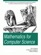Mathematics for Computer Science (Eric Lehman, et al)

This book covers elementary discrete mathematics for computer science and engineering. It emphasizes mathematical definitions and proofs as well as applicable methods. explores the topics of basic combinatorics, number and graph theory, logic and proof techniques.

•Advances in Discrete Differential Geometry (Alexander I. Bobenko)

It surveys the fascinating connections between discrete models in differential geometry and complex analysis, integrable systems and applications in computer graphics and is illustrated to convince readers it's both beautiful and useful.

•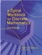A Spiral Workbook for Discrete Mathematics (Harris Kwong)

This is a book on discrete mathematics with an emphasis on motivation. It explains and clarifies the unwritten conventions in mathematics, and guides the students through a detailed discussion on how a proof is revised from its draft to a final polished form.

•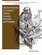Discrete Structures for Computer Science: Counting, Recursion

This book provides a broad introduction to some of the most fascinating and beautiful areas of discrete mathematical structures with examples in logic, applications of the principle of inclusion and exclusion and finally the pigeonhole principal.

•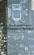Applied Discrete Structures, Fundamentals (Al Doerr, et al)

This book contains most of the fundamental concepts taught in a one semester course in discrete mathematics which is a required course for students in Computer Science, Mathematics and Information Technology.

•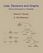Lists, Decisions and Graphs - With an Introduction to Probability

In this book, four basic areas of discrete mathematics are presented: Counting and Listing (Unit CL), Functions (Unit Fn), Decision Trees and Recursion (Unit DT), and Basic Concepts in Graph Theory (Unit GT).

•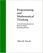Programming and Mathematical Thinking: Discrete Math & Python

Starting at an elementary level, this book teaches about fundamental structures of discrete mathematics and many simple but powerful programming techniques using those structures.

•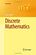Discrete Mathematics for Computer Science (Jean Gallier)

This book gives an introduction to discrete mathematics for beginning undergraduates. One of original features of this book is that it begins with a presentation of the rules of logic as used in mathematics. Many examples of formal and informal proofs are given.

•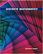Discrete Mathematics for Computer Science (Kenneth P. Bogart)

This book gives thorough coverage to topics that have great importance to computer scientists and provides a motivating computer science example for each math topic. Contents: Counting; Cryptography and Number Theory; Reflections on Logic and Proof.

•Exploring Discrete Mathematics With Maple (Kenneth H. Rosen)

This is is a supplement in discrete mathematics to concentrate on the computational aspects of the computer algebra system Maple. Detailed instructions for the use of Maple are included in an introductory chapter and in each subsequent chapter.

•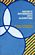Discrete Mathematics with Algorithms (M. O. Albertson, et al)

This is a first-year course in discrete mathematics requireing no calculus or computer programming experience.

•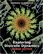Exploring Discrete Dynamics (Andrew Wuensche)

This book is a comprehensive guide to studying cellular automata and discrete dynamical networks with the classic software Discrete Dynamics Laboratory (DDLab), widely used in research and education.

•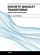Discrete Wavelet Transforms - Theory and Applications

The present book describes the latest progress in discrete wavelet transform (DWT) analysis in non-stationary signal processing, multi-scale image enhancement as well as in biomedical and industrial applications.

•Discrete Wavelet Transforms - Algorithms and Applications

This book reviews the recent progress in discrete wavelet transform (DWT) algorithms and applications. The book covers a wide range of methods for constructing DWTs.

•Mathematics Of The Discrete Fourier Transform (DFT)

Detailed derivation of the Discrete Fourier Transform (DFT) and its associated mathematics, including elementary audio signal processing applications and matlab programming examples.

•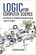Logic for Computer Science: Automatic Theorem Proving

This book introduces mathematical logic with an emphasis on proof theory and procedures for algorithmic construction of formal proofs. It is useful for the formalization of proofs and basics of automatic theorem proving.

•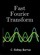Fast Fourier Transforms (C. Sidney Burrus, et al)

This book focuses on the Discrete Fourier Transform (DFT), discrete convolution, and, particularly, the fast algorithms to calculate them. Topics on digital signal processing since its beginning, and new results in hardware, theory and applications, etc.

•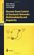Discrete-Event Control of Stochastic Networks (Eitan Altman, et al)

Opening new directions in research in both discrete event dynamic systems as well as in stochastic control, this volume focuses on a wide class of control and of optimization problems over sequences of integer numbers.

•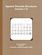Applied Discrete Structures (Ken Levasseur, et al)

This book is an undergraduate textbook in discrete mathematics, focusing on the structural properties of mathematical objects. These include matrices, functions, graphs, trees, lattices and algebraic structures.

•Discrete Oscillation Theory (Ravi P. Agarwal, et al)

This book is devoted to a rapidly developing branch of the qualitative theory of difference equations with or without delays. It presents the theory of oscillation of difference equations, exhibiting classical as well as very recent results in that area.

•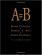A=B (Marko Petkovsek, Herbert S. Wilf, Doron Zeilberger)

This book is of interest to mathematicians and computer scientists working in finite mathematics and combinatorics. It presents a breakthrough method for analyzing complex summations.

•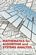Mathematics for Algorithm and Systems Analysis (E. A. Bender)

This up-to-date textbook assists undergraduates in mastering the ideas and mathematical language to address problems that arise in the field's many applications.

•Art Gallery Theorems and Algorithms (Joseph O'Rourke)

This book is the most comprehensive collection of results on polygons currently available and thus earns its place as a standard text in discrete and computational geometry. It is very well written and a pleasure to read.

•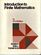Introduction to Finite Mathematics (John G. Kemeny, et al)

The goal of this book is for the students mathematical maturity and appreciation for the usefulness of mathematics to grow simultaneously.

•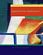Applied Finite Mathematics (Roberta Bloom)

This book demonstrates how mathematics applies to various fields of study, packed with real data and real-life applications. It uses a large number of applications based on real data to help you see how mathematics relates to your own life and interests.

•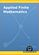Applied Finite Mathematics (Rupinder Sekhon)

This book covers topics including linear equations, matrices, linear programming (geometrical approach and simplex method), the mathematics of finance, sets and counting, probability, Markov chains, and game theory.

•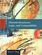Discrete Structures, Logic, and Computability (James L. Hein)

This book contains programming experiments that are designed to reinforce the learning of discrete mathematics, logic, and computability.

•Prolog Experiments in Discrete Math, Logic, and Computability

This book contains programming experiments that are designed to reinforce the learning of discrete mathematics, logic, and computability.

•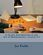A Gentle Introduction to the Art of Mathematics (David Winterfeldt)

The purpose of this book is to acclimatize the student to some of the culture and terminology of mathematics and to begin developing in them a proficiency at reading and writing mathematical proofs.

•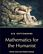Six Septembers: Mathematics for the Humanist (Patrick Juola)

Developing the skills to enable humanist scholars to address complicated technical material with confidence. Mathematics operates under a regime of shared assumptions, and it is our purpose to elucidate some of those assumptions for the newcomer.

•Concrete Mathematics: A Foundation for Computer Science

This book introduces the mathematics that supports advanced computer programming and the analysis of algorithms. The primary aim of its well-known authors is to provide a solid and relevant base of mathematical skills.

•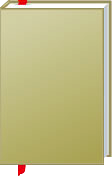Discrete and Finite Mathematics

This is the previous page of Discrete and Finite Mathematics, we are in the processing to convert all the books there to the new page. Please check this page again!!!

Book CategoriesAll CategoriesRecent BooksIT Research LibraryMiscellaneous Books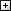Computer LanguagesComputer ScienceData Science/DatabasesElectronic EngineeringJava and Java EE (J2EE)Linux and UnixMathematicsMicrosoft and .NETMobile ComputingNetworking and CommunicationsSoftware EngineeringSpecial TopicsWeb Programming
Other Categories